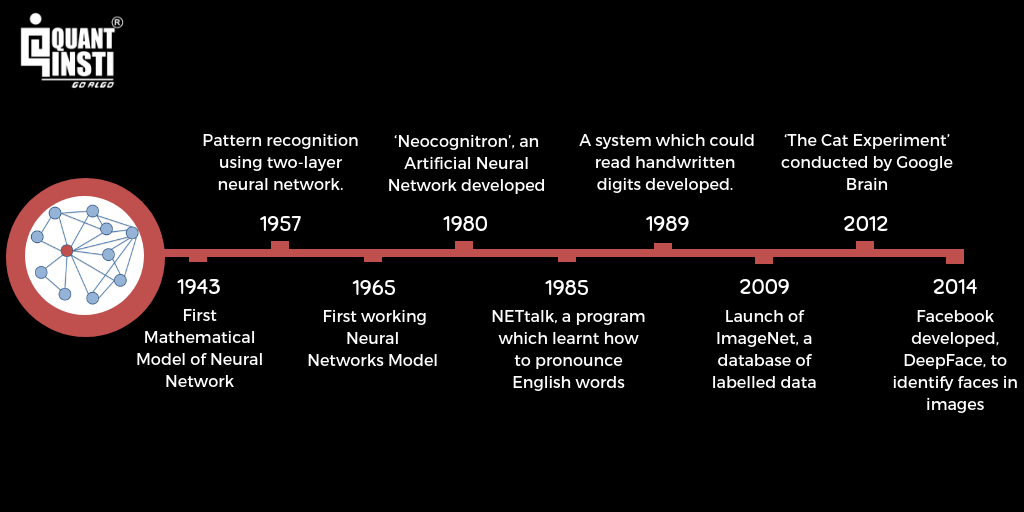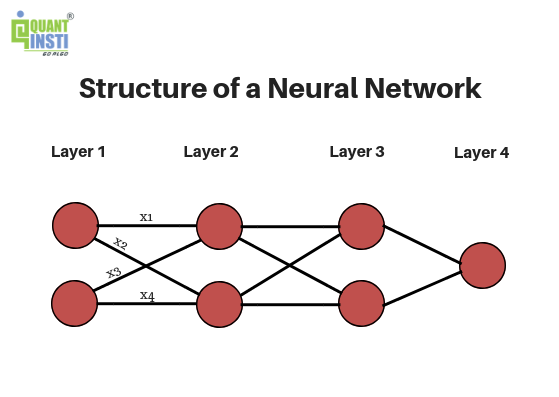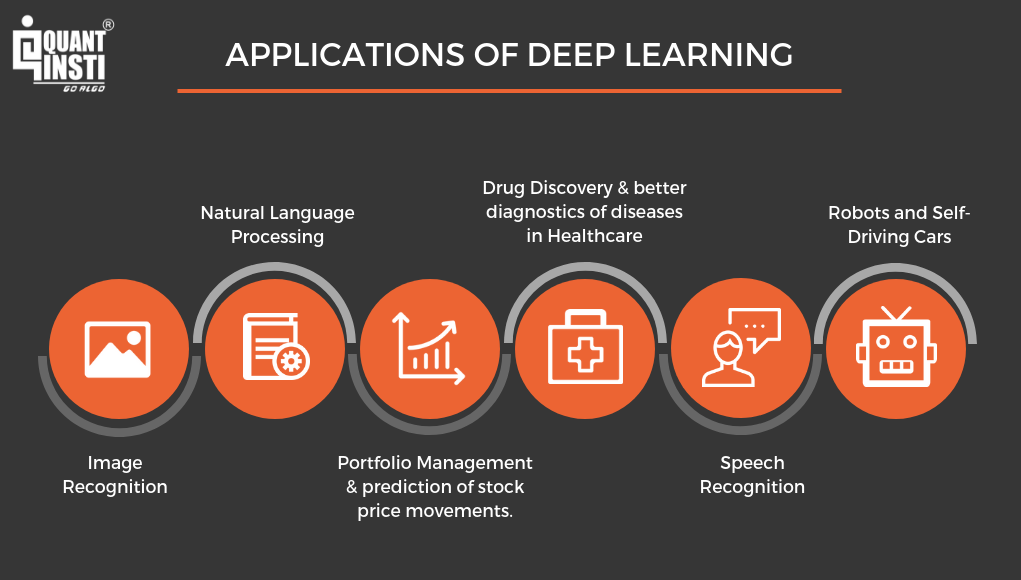# Introduction To Deep Learning And Neural Network

“Artificial intelligence would be the ultimate version of Google. The ultimate search engine that would understand everything on the web. It would understand exactly what you wanted, and it would give you the right thing”, said Larry Page, CEO of Alphabet, in 2000 when most of us considered Google to be just a simple search engine. Today, artificial intelligence and deep neural network learning are making things possible which a few years ago, were even hard to imagine!

Thanks to fields like Machine Learning and Deep Learning, which are a part of Artificial Intelligence, we have been able to automate and integrate ‘learning’ within machines.

In this article on Introduction to Deep Learning, we will be learning what Deep Learning is and how it is being used in the field of Artificial Intelligence.

The contents of this article on "Introduction to Deep Learning and Neural Network" are as follows:

###What is Deep Learning?

Deep Learning is a Machine Learning method involving the use of Artificial Deep Neural Network. Just as the human brain consists of nerve cells or neurons which process information by sending and receiving signals, the deep neural network learning consists of layers of ‘neurons’ which communicate with each other and process information.

The ‘deep’ in Deep Learning points out to the number of layers within the network; more the number of layers, deeper is the network.

Deep neural network can work with labelled data as well as unlabelled data which allows for both supervised and unsupervised learning. However, a large amount of data is required to train the deep neural network which may help in producing more accurate results.

These networks are also capable of recognizing the errors or loss in the output and correcting them without the requirement of human intervention.

###History of Deep Learning

Along with the introduction to deep learning and understanding what it is, we will also look into how it all started. As we now know, deep learning networks are basically deep neural network with many layers.

The first mathematical model of a neural network was created by Walter Pitts and Warren McCulloch in 1943 which demonstrated the thought process of the human brain. From here began the journey of deep neural network and deep learning.

This journey can be represented as below:• 1957 - Frank Rosenblatt submitted a paper titled ‘The Perceptron: A Perceiving and Recognizing Automaton’, which consisted of an algorithm or a method for pattern recognition using a two-layer neural network.
• 1965 - Alexey Ivakhnenko and V.G. Lapa developed the first working neural network and Alexey Ivakhnenko created an 8-layer deep neural network in 1971 which was demonstrated in the computer identification system, Alpha. This was the actual introduction to deep learning.
• 1980 - Kunihiko Fukushima developed the ‘Neocognitron’, an artificial deep neural network with multiple and convolutional layers to recognize visual patterns.
• 1985 - Terry Sejnowski created NETtalk, a program which learnt how to pronounce English words.
• 1989 - Yann LeCun, using convolution deep neural network, developed a system which could read handwritten digits.
• 2009 - As deep learning models require a tremendous amount of labelled data to train themselves in supervised learning, Fei-Fei Li launched ImageNet, which is a large database of labelled images.
• 2012 - The results of ‘The Cat Experiment’ conducted by Google Brain were released. This experiment was based on unsupervised learning in which the deep neural network worked with unlabelled data to recognize patterns and features in the images of cats. However, it could only recognize 15% of images correctly.
• 2014 - Facebook developed, DeepFace, a deep learning system to identify and tag faces of users in the photographs.

###Difference between Machine Learning and Deep Learning

Deep Learning is actually a subset of Machine Learning, hence often the two terms are confused by people. However, they differ in their capabilities. That is why it is necessary to know about machine learning while reading about the introduction to deep learning.

Machine Learning models lack the mechanism to identify errors, in such cases the programmer needs to step in to tune the model for more accurate decisions, whereas deep learning models can identify the inaccurate decision and correct the model on its own without human intervention.

But for doing so, deep learning models require a huge amount of data and information, unlike Machine Learning models.

###Working of Deep Neural Network

Until now we have understood the basics and introduction to deep learning, and now its time to know about how the deep neural network works.

As explained earlier, deep neural network gets its name due to a high number of layers in the networks. Let us now understand what these layers are and how are they used in the deep neural network to give a final output by referring to the diagram given below:#### Layers in Deep Neural Network

By looking at this diagram, we see that there are 4 layers present in this deep neural network namely Layer 1, Layer 2, Layer 3 and Layer 4. Every deep neural network consists of three types of layers, which are:

Input Layer (Layer 1): This layer is the first layer in a  deep neural network and it provides the input parameters required to process the information. It simply passes these parameters to the further layers without any computation at this layer.

Hidden Layers (Layers 2 and 3): These layers in the deep neural network perform the necessary computations on the inputs received from the previous layers and pass on the result to the next layer. It is crucial to decide the number of layers and the number of neurons in each layer so as to increase the efficiency of the deep neural network. More the number of hidden layers, deeper is the network.

Output Layer (Layer 4): This layer in the deep neural network gives us the final output after receiving the results from the previous layers.

Now that we have understood the types of layers present in a network, let's learn how these layers actually function and give the output data.

Each neuron is connected to all the neurons in the next layer and all these connections have some weights associated with them. But what are these weights and why are they used?

#### Weights in Deep Neural Network

Weights, as the name suggests, are used to attach some weightage to a certain feature. Some features might be more important than other features to get the desired output.

For example, close prices and SMAs of the previous days will be considered as more important features than high or low prices while predicting the stock prices for the next day, this will affect the weights attached to these parameters.

These weights are used to calculate the weighted sum for each neuron. x1, x2, x3, x4 represent the weights associated with the corresponding connections in the deep neural network.

Along with the weights, each hidden layer has an activation function associated with it.

#### Activation Function in Deep Neural Network

Activation functions decide whether a neuron should be activated or not based on their weighted sum. These are also used to introduce non-linearity by using functions like sigmoid and tanh thus allowing computations for more complex tasks. Without the activation function, the deep neural network would act as a simple linear regression model.

Here are examples of a few activation functions which are used:

• Tanh: Avoids bias in gradients
• Rectified Linear Unit (ReLU): Used for Image Processing
• Softmax: To retain relevance of outliers

In addition to this, we also add a ‘bias’ neuron to each layer to enable moving the activation function along the x-axis to the left or to the right thus allowing us to fit the activation function better. The bias term which is a constant term also acts as an output whenever the input is absolute zero.

#### Processing of Deep Neural Network

The processing starts by calculating the weighted sums for each neuron in the first hidden layer using the inputs received from the input layer. The weighted sums are the sum of the products of the input with the corresponding weights for each connection.

The activation function corresponding to each layer then acts upon these weighted sums to give a final output. This process can also be known as forward propagation.

Once the processing is completed, the predicted output is compared with actual output to determine the error or loss. For a deep neural network to work accurately, this loss function must be minimized so that the predicted output is as close to the actual output as possible. As we initially choose random weights for the connections in the deep neural network, they might not be the best choice.

Hence, to minimize the loss function, we need to adjust the weights and biases to get accurate results. Backpropagation is the process used to tune the weights and biases such that we get the optimal values of weights and biases thus giving us higher accuracy in our results.

###Deep Learning ApplicationsThis blog on 'Introduction to Deep Learning' covered the definitions, difference, history, and applications of Deep Learning and helped us understand how artificial deep neural network functions.

Now, that we are familiar with the introduction to deep learning, we can move ahead to acquire more knowledge about it. You can also learn how to use deep learning and neural network for trading using Python in the below course.

You can enroll for the neural network tutorial on Quantra where you can use advanced neural network techniques and latest research models such as LSTM & RNN to predict markets and find trading opportunities. Keras, the relevant python library is used.